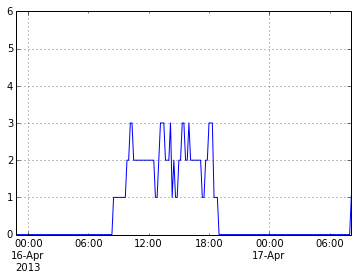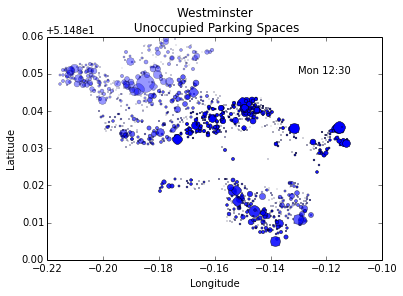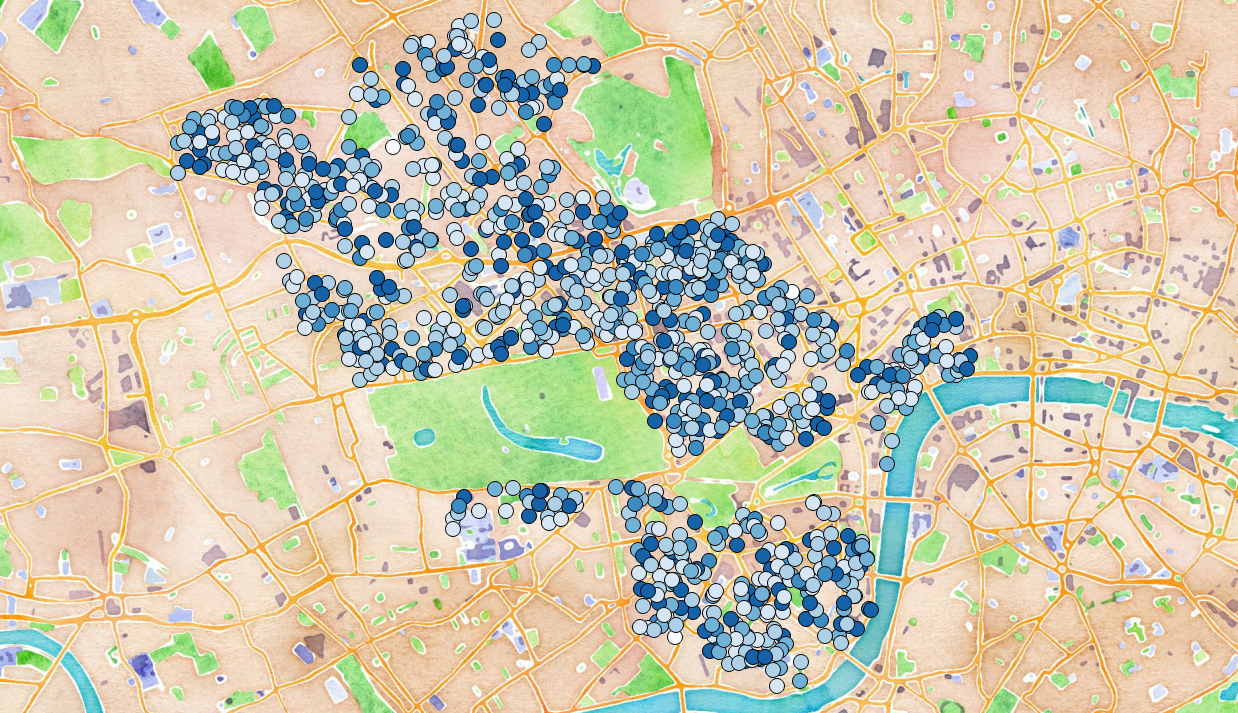In :
import pandas as pd
from pandas import Series, DataFrame, Panel
import numpy as np
import datetime
import matplotlib.pyplot as plt
%matplotlib inline

In :
COLUMN_NAMES = ["amount paid", "paid duration mins", "start date", \
"start day", "end date", "end day", "start time", "end time", \
"DesignationType", "Hours of Control", "Tariff", "Max Stay", \
"Spaces", "Street", "x coordinate", "y coordinate", "latitude", \
"longitude"]
PARKING_DATA = 'ParkingCashlessDenorm.csv'

In :
raw_data = pd.read_csv(PARKING_DATA, names=COLUMN_NAMES, header=None)

Out:
amount paid paid duration mins start date start day end date end day start time end time DesignationType Hours of Control Tariff Max Stay Spaces Street x coordinate y coordinate latitude longitude
0 3.3 45 2013-02-06 00:00:00 Wednesday 2013-02-06 00:00:00 Wednesday 10:58 11:43 Shared Use P&D Mon - Sat 8.30am - 6.30pm 4.4 4 hours 13 Hyde Park Square 527154.134706 181061.021077 51.514183 -0.168964
1 4.4 60 2013-02-06 00:00:00 Wednesday 2013-02-06 00:00:00 Wednesday 12:44 13:44 Shared Use P&D Mon - Sat 8.30am - 6.30pm 4.4 4 hours 13 Hyde Park Square 527154.134706 181061.021077 51.514183 -0.168964
2 2.4 60 2013-02-06 00:00:00 Wednesday 2013-02-06 00:00:00 Wednesday 13:44 14:44 P&D + PbP Mon - Fri 8.30-6.30 Sat 8.30 - 1.30 2.4 4 Hours 13 Queensborough Terrace 526036.819680 180730.025823 51.511458 -0.185176
3 2.4 60 2013-06-08 00:00:00 Saturday 2013-06-08 00:00:00 Saturday 08:43 09:43 P&D + PbP Mon - Sat 8.30am - 6.30pm 2.4 4 Hours 15 Winsland Street 526709.888753 181367.836585 51.517040 -0.175253
4 7.0 105 2013-06-08 00:00:00 Saturday 2013-06-08 00:00:00 Saturday 15:44 17:29 P&D + PbP Mon - Sat 8.30am - 6.30pm 4 4 hours 5 Wigmore Street 528210.089071 181233.842719 51.515498 -0.153692
In :
# pandas will usually do a good job of interpretting time and date information.
# However, rather annoyingly the date and time data has been stored in separate columns
# so we need to do a bit of work to get consistent timestamps to work with.

# First we convert the date information into timestamp format
# Since no time information is given pandas assumes the time as 00:00:00
raw_data['start date'] = pd.to_datetime(raw_data['start date'])
raw_data['end date'] = pd.to_datetime(raw_data['end date'])

# Next convert the time information into timestamp format
# Since the time information has no date associated with it pandas assumes
# the date is today, so we'll need to subtract today's date to get the time on its own
raw_data['start time'] = pd.to_datetime(raw_data['start time'])
raw_data['end time'] = pd.to_datetime(raw_data['end time'])

# We can now construct a proper timestamp for each of the records by adding
# the date and time and subtracting today's date
ts_today = pd.to_datetime('00:00:00')
raw_data['start time'] = raw_data['start time'] - ts_today
raw_data['end time'] = raw_data['end time'] - ts_today
raw_data['start datetime'] = raw_data['start date'] + raw_data['start time']
raw_data['end datetime'] = raw_data['end date'] + raw_data['end time']

In [ ]:
# i've subsequently realised that the whole of the above code can be replaced with this
names=COLUMN_NAMES,
parse_dates={'end datetime': ['end date','end time'] , 'start datetime':['start date','start time']})

# How nice is that!

In :
# Now we want to group together all records for each parking location based on
# the latitude and longitude, the 'Street' is ambiguous since some streets have
# more than one parking location
parking_locations = raw_data.groupby(by = ['latitude', 'longitude'])

# get a single location
df1 = raw_data.ix[parking_locations.indices[parking_locations.indices.keys()]]

arrivals = Series(np.ones(df1.shape), index = df1['start datetime'])
departures = Series(-1*np.ones(df1.shape), index = df1['end datetime'])
arr_dep = arrivals.append(departures)
occupancy = arr_dep.resample('10Min', how ='sum').fillna(0).cumsum()
occupancy.plot(xlim =(occupancy.index,occupancy.index))

Out:
<matplotlib.axes.AxesSubplot at 0x3da5f470>In [ ]:
# We now construct a Panel object containing the average occupancy for each day of the week
first_time = 1
for j, k in enumerate(parking_locations.indices.keys()):
# print j
# look at one location at a time
df1 = raw_data.ix[parking_locations.indices[k]]
# Calculate occupancy by incrementing a count for every arrival and decrementing the
# same count for every departure
arrivals = Series(np.ones(df1.shape), index = df1['start datetime'])
departures = Series(-1*np.ones(df1.shape), index = df1['end datetime'])
occupancy = arrivals.append(departures)
occupancy = occupancy.resample('10Min', how = 'sum').fillna(0).cumsum()

# split the data by day of the week
mon = occupancy.ix[occupancy.index.dayoftheweek == 0]
tue = occupancy.ix[occupancy.index.dayoftheweek == 1]
wed = occupancy.ix[occupancy.index.dayoftheweek == 2]
thu = occupancy.ix[occupancy.index.dayoftheweek == 3]
fri = occupancy.ix[occupancy.index.dayoftheweek == 4]

df2 = DataFrame(mon.groupby(mon.index.time).mean(), columns =['Monday'])
df2['Tuesday']=tues.groupby(tues.index.time).mean()
df2['Wednesday']=weds.groupby(weds.index.time).mean()
df2['Thursday']=thur.groupby(thur.index.time).mean()
df2['Friday']=fri.groupby(fri.index.time).mean()
# store some other information for each location for ease of access
df2['Street'] = df['Street'].values
df2['Spaces'] = df['Spaces'].values
df2['Tariff'] = df['Tariff'].values

# Add this newly created DataFrame containing occupancy information to a Panel object
if first_time:
first_time = 0
p = Panel(data = [df2.values], items = [k], major_axis = df2.index, minor_axis = df2.columns)
else:
p[k] = df2

In :
# save/load the panel object
# pd.save(p,'parking_data_panel.pkl')
p

Out:
<class 'pandas.core.panel.Panel'>
Dimensions: 1342 (items) x 1440 (major_axis) x 8 (minor_axis)
Items axis: (51.51394676, -0.14329589) to (51.5202289, -0.14500824)
Major_axis axis: 00:00:00 to 23:59:00
Minor_axis axis: Monday to Tariff
In :
# We can now interrogate the panel object to look at different aspects of the data
# let's look at the number of free spaces at 12:30 for an average monday

h, m = 12, 30
# get the total number of spaces at each location
spaces = p.minor_xs( u'Spaces')
mon_occupancy = p.minor_xs( u'Monday')
mon_free_spaces = spaces - mon_occupancy

# Use the Tariff to set an alpha value so we can see which parking locations are more expensive
tariff = p.minor_xs( u'Tariff').ix[datetime.time(h,m)]

# get the free spaces for 12:30
free_space_0 = mon_free_spaces.ix[datetime.time(h,m)]

# plot free space for each location using a the size of a circle to indicate the number of free spaces
for tup in free_space_0.keys():
plt.plot(tup, tup, 'bo', ms = np.max([0,free_space_0[tup]/2]),alpha = (1 + float(tariff[tup]))/5.4)

plt.title('Westminster \n Unoccupied Parking Spaces')
plt.text(- 0.13, 51.53,'Mon ' + str(h).zfill(2) + ':' + str(m).zfill(2))
plt.xlabel('Longitude')
plt.ylabel('Latitude')

plt.show()In [ ]:
# Finally, we can loop through different times to create a series of images for creating an animation

# set the time step for visualising occupancy
ts = 5
cnt = 0
for h in np.arange(0,24):
for m in np.arange(0,60,ts):

# Use the Tariff to set an alpha value so we can see which parking locations are more expensive
tariff = p.minor_xs( u'Tariff').ix[datetime.time(h,m)]

# get the free spaces for 12:30
free_space_0 = mon_free_spaces.ix[datetime.time(h,m)]

# plot free space for each location using a the size of a circle to indicate the number of free spaces
for tup in free_space_0.keys():
plt.plot(tup, tup, 'bo', ms = np.max([0,free_space_0[tup]/2]),alpha = (1 + float(tariff[tup]))/5.4)

plt.title('Westminster \n Unoccupied Parking Spaces')
plt.text(- 0.13, 51.53,'Mon ' + str(h).zfill(2) + ':' + str(m).zfill(2))
plt.xlabel('Longitude')
plt.ylabel('Latitude')

cnt = cnt + 1
fname = 'image' + str(cnt).zfill(5) + '.png'

#print fname

plt.savefig(fname)
plt.clf()

In :
from IPython.display import YouTubeVideo

# QGIS (visualisation of shapefiles backed by OpenLayers maps from Google and OSM)